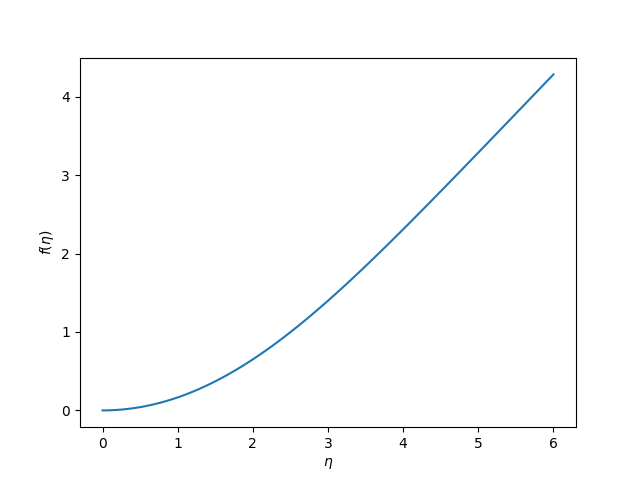## Solving the Blasius equation

| categories: bvp | tags:

In fluid mechanics the Blasius equation comes up (http://en.wikipedia.org/wiki/Blasius_boundary_layer) to describe the boundary layer that forms near a flat plate with fluid moving by it. The nonlinear differential equation is:

\begin{eqnarray} f''' + \frac{1}{2} f f'' &=& 0 \\ f(0) &=& 0 \\ f'(0) &=& 0 \\ f'(\infty) &=& 1 \end{eqnarray}

This is a nonlinear, boundary value problem. The point of solving this equation is to get the value of $$f''(0)$$ to evaluate the shear stress at the plate.

We have to convert this to a system of first-order differential equations. Let $$f_1 = f$$, $$f_2 = f_1'$$ and $$f_3 = f_2'$$. This leads to:

\begin{eqnarray} f_1' = f_2 \\ f_2' = f_3 \\ f_3' = -\frac{1}{2} f_1 f_3 \\ f_1(0) = 0 \\ f_2(0) = 0 \\ f_2(\infty) = 1 \end{eqnarray}

It is not possible to specify a boundary condition at $$\infty$$ numerically, so we will have to use a large number, and verify it is "large enough". From the solution, we evaluate the derivatives at $$\eta=0$$, and we have $$f''(0) = f_3(0)$$.

We have to provide initial guesses for f_1, f_2 and f_3. This is the hardest part about this problem. We know that f_1 starts at zero, and is flat there (f'(0)=0), but at large eta, it has a constant slope of one. We will guess a simple line of slope = 1 for f_1. That is correct at large eta, and is zero at η=0. If the slope of the function is constant at large $$\eta$$, then the values of higher derivatives must tend to zero. We choose an exponential decay as a guess.

Finally, we let a solver iteratively find a solution for us, and find the answer we want. The solver is in the pycse module.

import numpy as np
from pycse import bvp

def odefun(F, x):
f1, f2, f3 = F.T
return np.column_stack([f2,
f3,
-0.5 * f1 * f3])

def bcfun(Y):
fa, fb = Y[0, :], Y[-1, :]
return [fa,        # f1(0) =  0
fa,        # f2(0) = 0
1.0 - fb]  # f2(inf) = 1

eta = np.linspace(0, 6, 100)
f1init = eta
f2init = np.exp(-eta)
f3init = np.exp(-eta)

Finit = np.column_stack([f1init, f2init, f3init])

sol = bvp(odefun, bcfun, eta, Finit)
f1, f2, f3 = sol.T

print("f''(0) = f_3(0) = {0}".format(f3))

import matplotlib.pyplot as plt
plt.plot(eta, f1)
plt.xlabel('$\eta$')
plt.ylabel('$f(\eta)$')
plt.savefig('images/blasius.png')

f''(0) = f_3(0) = 0.3324911095517483<2017-05-17 Wed> You need pycse 1.6.4 for this example.